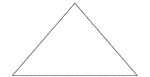### Isosceles Triangle degrees 81, 49.5, 49.5

An isosceles triangle with angles 81, 49.5, 49.5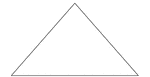### Isosceles Triangle degrees 82, 49, 49

An isosceles triangle with angles 82, 49, 49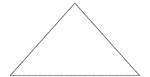### Isosceles Triangle degrees 83, 48.5, 48.5

An isosceles triangle with angles 83, 48.5, 48.5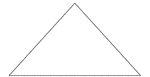### Isosceles Triangle degrees 84, 48, 48

An isosceles triangle with angles 84, 48, 48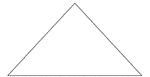### Isosceles Triangle degrees 85, 47.5, 47.5

An isosceles triangle with angles 85, 47.5, 47.5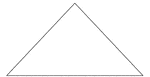### Isosceles Triangle degrees 86, 47, 47

An isosceles triangle with angles 86, 47, 47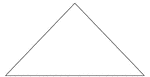### Isosceles Triangle degrees 87, 46.5, 46.5

An isosceles triangle with angles 87, 46.5, 46.5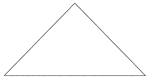### Isosceles Triangle degrees 88, 46, 46

An isosceles triangle with angles 88, 46, 46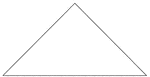### Isosceles Triangle degrees 89, 45.5, 45.5

An isosceles triangle with angles 89, 45.5, 45.5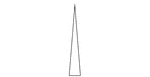### Isosceles Triangle degrees 9, 85.5, 85.5

An isosceles triangle with angles 9, 85.5, 85.5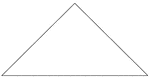### Isosceles Triangle degrees 90, 45, 45

An isosceles triangle with angles 90, 45, 45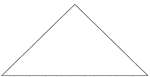### Isosceles Triangle degrees 91, 44.5, 44.5

An isosceles triangle with angles 91, 44.5, 44.5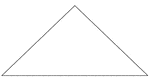### Isosceles Triangle degrees 92, 44, 44

An isosceles triangle with angles 92, 44, 44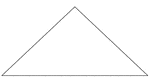### Isosceles Triangle degrees 93, 43.5, 43.5

An isosceles triangle with angles 93, 43.5, 43.5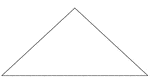### Isosceles Triangle degrees 94, 43, 43

An isosceles triangle with angles 94, 43, 43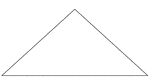### Isosceles Triangle degrees 95, 42.5, 42.5

An isosceles triangle with angles 95, 42.5, 42.5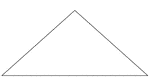### Isosceles Triangle degrees 96, 42, 42

An isosceles triangle with angles 96, 42, 42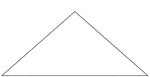### Isosceles Triangle degrees 97, 41.5, 41.5

An isosceles triangle with angles 97, 41.5, 41.5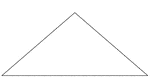### Isosceles Triangle degrees 98, 41, 41

An isosceles triangle with angles 98, 41, 41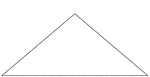### Isosceles Triangle degrees 99, 40.5, 40.5

An isosceles triangle with angles 99, 40.5, 40.5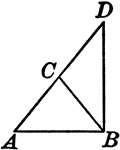### Leg Produced through the Vertex of an Isosceles Triangle

Illustration to show that if one of the legs of an isosceles triangle is produced through the vertex…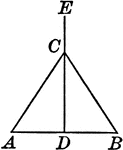### Perpendicular Bisector of the Base of an Isosceles Triangle

Illustration to show that the perpendicular bisector of the base of an isosceles triangle passes through…